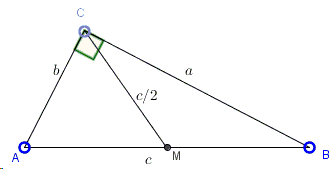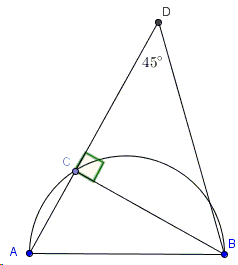# Problem 4 from the 1959 IMO: Construction of a Right Triangle

Here's a problem from an old Russian problem collection:

Construct a right-angled triangle whose hypotenuse $c$ is given if it is known that the median from the right angle equals the geometric mean of the remaining two sides of the triangles.SolutionThe applet below illustrates the problem and a solution:

The solution is based on the observation that the triangles $ABC$ and $CBD$ are similar, implying the proportion $\displaystyle\frac{b}{c}=\frac{h}{a}$. The solution further makes use of the fact that in a right triangle the midpoint of the hypotenuse serves as the circumcenter so that the median from the right angle equals half of the hypotenuse. The construction is then obvious: draw a line parallel to the hypotenuse at distance $c/4$ from it. The two points where that line intersects the semicircle with $AB$ as diameter are sought locations of vertex $C$.

The original solution [The IMO Compendium, p357-358] went in a somewhat different way:

By the Pythagorean theorem $c^{2}=a^{2}+b^{2}$ which, together with $c/2=\sqrt{ab}$, implies $a+b=c\sqrt{3/2}$. Given a desired $\Delta ABC$, let $D$ be a point on $AC$ such that $CD=CB$. In that case, $AD=a+b=c\sqrt{3/2}$, and also $\angle ADB=45^{\circ}$, because $BC=CD$.With this analysis in mind, measure $AD=c\sqrt{3/2}$ and draw a ray $DX$ such that $\angle ADX=45^{\circ}$. Next, construct circle $C(A,c)$ with center $A$ and radius $c$. Take $B$ to be the point of intersection of the circle and $DX$ and drop a perpendicular $BC$ to $AD$.

### References

1. D. Djukic et al, The IMO Compendium, Springer, 2011 (Second edition)